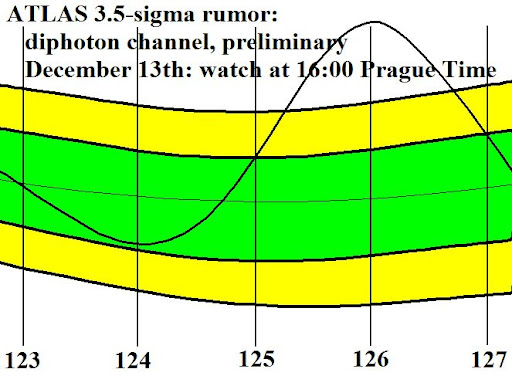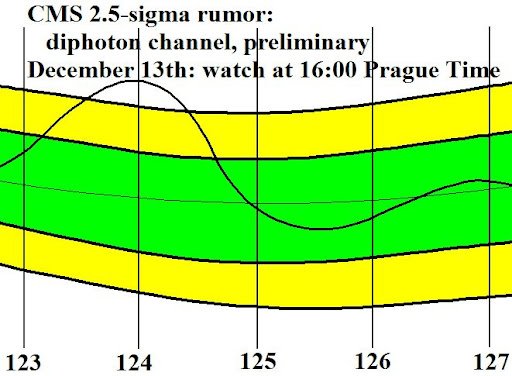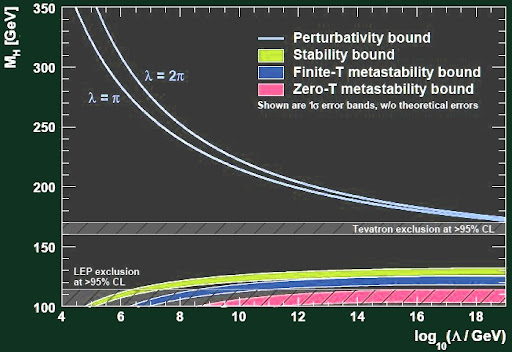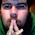## Wednesday, December 07, 2011

### Higgs at 125 GeV and SUSY with heavy scalars

The world is eagerly expecting whether or not CERN will announce some evidence from the diphoton channel that there is a Higgs boson whose mass is between 124 and 126 GeV.Well, the bump will probably be a bit wider. The image should say 14:00, not 16:00, as the beginning of the CERN webcast. Sorry for the error in the timing, I can't edit the image file anymore (except for wasting 5 more minutes).

I will talk about a 125 GeV Higgs, assuming that the answer to the previous question is Yes and that the two nearby bumps seen by CMS and ATLAS are signs of the same thing (the width of the Higgs bump in the diphoton channel should be close to 2 GeV, too).IQ test: Why is this damn Rubik cube posted in an article about a Higgs at 125 GeV?

In my previous Higgs blog entry, I have already sketched some hidden messages that a Higgs boson of this mass could be trying to communicate to us. The viXra blog has said a few complementary things as well.

Let me expand on the topic what the different Higgs masses could mean.

First, as argued in a reblog by Flip Tanedo, among other places on TRF, the electroweak theory needs "something like the Higgs" for the particles including W-bosons and Z-bosons to get massive in a friendly way. Vector particles, i.e. spin-one particles with an "polarization arrow" attached to them must be analogous to photons which are particles of the electromagnetic radiation.The $$H\to \gamma\gamma$$ decay of the Higgs bosons where the near-discovery of the Higgs should be announced next Tuesday boils down to the Feynman diagrams (histories of splitting and merging elementary particles) above. There is no tree-level (free-of-loops) diagram coupling $$H\gamma\gamma$$ because the Higgs is electrically neutral and therefore doesn't interact with the electromagnetic field at the level of classical physics: the Higgs can't decay to two photons "directly". However, with a single loop (quantum contribution involving virtual particles) of W-bosons or top-quarks, you may get a pretty large amplitude, anyway. Note that both triangles (like for the top-quark) and a circle is allowed for the W-bosons because both cubic (3 external legs) and quartic (4 external legs) interaction vertices between the W-bosons and photons exist. Apologies for the low color contrast. Via Resonaances

Eating Higgs components to gain new polarizations

As you know, the electromagnetic waves are transverse so they remember a direction of the electric field that is perpendicular to the direction of the photon's motion. The transverse character of the photons is closely linked to the photon's being spin-one (vector) particles. Spin-zero (scalar) particles would have to be associated with longitudinal waves (such as sound, even though sound in the air can't be easily described as a stream of "sonic" particles or "phonons" because the air is too chaotic or "incoherent").The timing should say 14:00.

There are two possible transverse polarizations of a photon. If it is flying in the $$z$$ direction, the polarization may be along $$x$$ or $$y$$ axes. All other possibilities (including the circular ones) are complex linear combinations of these two. We say that in 3+1 dimensions, a massless vector particle (photon) has 2 polarizations. In $$d$$ spacetime dimensions, that would be $$d-2$$ polarizations: the temporal and longitudinal spatial polarizations (two in total) are unphysical.

The W-bosons and Z-bosons are massive, as we know, but they must still fundamentally interact in ways similar to the photon. Their being massive means that each such a particle has its preferred rest frame. In the rest frame, it's clear from the rotational symmetry that they must have 3 polarizations $$x,y,z$$ instead of just 2 of them.

The new polarization has to come from a new field – a scalar component of the Higgs field (doublet) that is eaten by the W-boson or Z-boson field. The only way to add such scalar "food from the fields" that keep the Lagrangian polynomial is to add a whole Higgs doublet $$H$$ (or several of them). It has two complex components: the three real "angular" coordinates labeling this doublet are the scalars that are "eaten" by the neutral Z-boson and the positive and negative W-boson. However, there's still the "absolute value" of $$H$$ or the radial coordinate of the Higgs doublet in the four-dimensional real space – and this is the new particle known as the Higgs boson.

Higgs self-interaction

If we start with the description of the W-bosons and Z-bosons that preserves the underlying $$SU(2)\times U(1)_Y$$ symmetry, where the first factor is an "electroweak isospin" and the second one is the "hypercharge" (the average electric charge in an isospin multiplet, times two, to follow the usual conventions), the role of the Higgs doublet $$H$$ is to break this symmetry down to $$U(1)_{EM}$$, the electromagnetic gauge symmetry we have known since the 19th century.

If the breaking didn't occur, i.e. if the vacuum had $$H=0$$, the W-bosons and Z-bosons would be as massless as the photons. Moreover, all the fermions would have to be massless as well. Electrons would be de facto indistinguishable from the neutrinos and upper quarks would be indistinguishable from the lower quarks. (Some generators of the greater unbroken gauge symmetry would be able to mix, i.e. to prove the physical indistinguishability of, particles of different electric charges: that's because some generators of the $$SU(2)$$ group don't commute with the electric charge $$Q$$ as operators.)

However, in the real vacuum around us, we have
$\langle H \rangle = v = 247\,\,{\rm GeV}.$ The expectation value of the Higgs – especially the radial component is a nonzero number. Because the kinetic term $$(\partial_\mu H)^2/2$$ in the Lagrangian has to have the dimension $$({\rm mass})^4$$, we see that both $$\partial_\mu$$ and $$H$$ must have the dimension of mass or energy, too. That's why the expectation value is expressed as a particular energy. The Higgs vacuum expectation value (vev) around 247 GeV defines the so-called electroweak scale: you see it's comparable to the W-boson and Z-boson masses near 80 and 90 GeV, respectively. It's no coincidence: these masses arise from the gauge bosons' interactions with $$H$$ (i.e. the interaction term is mostly multiplied by $$v$$) and the extra coupling constants $$g$$ in these terms aren't too far from $$1$$.

Why does Nature choose to keep the nonzero 247 GeV value of the Higgs boson's field and how does it preserve the nonzero value, so that the value of the Higgs field at each point doesn't roll to any other number, in particular to $$H=0$$? Nature probably chooses a simple solution: it postulates a potential energy that depends on $$H$$ and that punishes all values of $$H$$ whose absolute value is very different from those 247 GeV. The renormalizable potentials have to be polynomials of at most quartic order, so in the classical approximation, the potential is
$V(H) = \frac{\lambda}{2} (H^\dagger H - v^2)^2.$ Here, if you don't know what the dagger $$\dagger$$ is (no, it doesn't mean that $$H$$ recently died), just imagine that $$H^\dagger H \sim H^2$$. You see that the potential above is only vanishing for $$|H|=v$$, otherwise it's positive. So Nature will spontaneously choose any value of the Higgs doublet that satisfies $$|H|=v$$ in order to save energy (without any need to replace Edison's light bulbs). As we mentioned, $$H$$ has basically four real components, so this leaves the three angular coordinates of $$H$$ undetermined: they parameterize a three-dimensional sphere. However, all of the solutions are related by the original $$SU(2)\times U(1)_Y$$ gauge transformations. Without a loss of generality, we may choose a particular component of $$H$$ to be the nonzero one, and make it real and positive. In the vacuum, we have
$\langle H \rangle = \left(\begin{array}{c} v\\0 \end{array} \right)$ The brackets on the left hand side represent the expectation values, i.e. the averaging over the quantum noise.The graph of the potential energy density $$V(H)$$ we are discussing here is known as the champagne bottle bottom potential in the first world (capitalist world of prosperity), Landau's buttocks potential in the second world (the former bloc of peace and socialism), and the Mexican hat potential in the third world. Note that the field $$H$$ at each point chooses one of the points at the minimum of the potential. All of them are physically equivalent a priori, because the fundamental equations of physics respect a symmetry that maps one such point into any other. However, the Higgs field's decision to pick one direction and discriminate against all others "spontaneously breaks" the $$SU(2)$$ symmetry and this is what makes most elementary particles (leptons, quarks, W-bosons, Z-bosons) massive and physically distinct from their $$SU(2)$$ partners (electron vs neutrino). Spontaneous breaking is just like the breaking of democracy in the Academia. A priori, the laws say that the right-wingers and left-wingers are equal. However, an extra field is introduced that forces everyone – without any rewriting of the laws – to label all right-wingers whom you dare to refer to "extremists" if you are a coward and a weasel like Michael Duff who is scared of perishing in the environment with a nonzero vev (political bias). The nonzero vev (political bias) is being preserved by a potential that makes the life of right-wingers more unpleasant and repels them from the system.

You must have noticed that there was an arbitrary positive constant $$\lambda$$ in front of the quartic potential. This "self-interaction" of the Higgs boson doesn't affect the preferred value of the Higgs field, $$H=v$$, but it does affect the Higgs mass. You may expand the potential around the $$H=v$$ minimum to see that
$V(H) = 2 \lambda v^2 (H-v)^2 + O\left((H-v)^3\right).$ The leading quadratic piece should be interpreted as an ordinary mass term
$V(H) = \frac{m_H^2}{2} (H-v)^2$ which relates the coefficients as
$4\lambda v^2 = m_H^2.$ Given a fixed and known $$v$$, the Higgs mass is an increasing function of the dimensionless coupling constant $$\lambda$$. So in my conventions, if the Higgs mass $$m_H$$ is equal to 125 GeV, we get almost exactly $$\lambda = 1/16$$. Don't get carried away by this numerology – equivalently, by the observation that 125 GeV is almost exactly one-half of the vev 247 GeV. Such an excitement would most likely be just childish numerology because as we will discuss soon, the value of $$\lambda$$ slightly depends on the mass scale where you use your physical theory. While the value $$1/16$$ was calculated by matching the low-energy parameters, the really fundamental value of $$\lambda$$ is one evaluated at high energies – which differs by logarithmic terms rooted in quantum mechanics – and this value of $$\lambda$$ may be very different from $$1/16$$.

Running $$\lambda$$ from the LHC scale to the Planck scale

As I discussed in the article about the possible divergence of the constant $$\lambda$$, this coupling constant, much like others, depends on the energy scale. Why is it so?

You may say that the claim that $$\lambda$$ is exactly dimensionless and $$H$$ has the dimension of the first power of mass etc. are just approximations. Quantum mechanics is allowed to correct many things and one of the things it corrects are the dimensions of fields and operators. So the coupling $$\lambda$$ is no longer "exactly" dimensionless, i.e. of dimension zero: it has a small fractional dimension that depends on the coupling constant itself. Similarly other operators have dimensions that are not quite integers as you would expect classically: they "run".

The infinitesimal correction to the dimension may always be represented as some kind of a "logarithmic running": try to differentiate $$E^{\Delta+\epsilon}$$ with respect to $$\epsilon$$ (which is what you do if you Taylor-expand the function for a small $$\epsilon$$) to see where the logarithms of the energy basically come from. It means that if you want to evaluate the value of the constant $$\lambda$$, or more precisely something like $$1/\lambda$$, at some energy scale $$E$$, it differs from the value at another energy scale $$E_0$$:
$\frac{1}{\lambda(E)} \sim \frac{1}{\lambda (E_0)} + K\cdot \ln(E/E_0).$ The coefficient $$K$$ determines the strength of the running; imagine it's smaller than one. Note that the logarithm is comparable to one (or a dozen) even if the energy scale ratio $$E/E_0$$ equals trillions or more. With some more refined normalizations and conventions, $$K$$ is known as the beta-function (this $$\beta$$ has no relationship to the same letter in $$\tan\beta$$). It may be either positive or negative. Before the early 1970s, people would think it's always positive but when they discovered QCD and "asymptotic freedom" (Gross, Wilczek, Politzer, thank you), theories with negative values became omnipresent.Yes, the gauge coupings run and so does the Higgs self-interaction. The running has the capacity to qualitatively change the character of physics at high energies relatively to what we believe has to occur at low energies. At high energies – somewhere between the LHC scale at a few TeV and the Planck energy of $$10^{19}$$ GeV – the coupling $$\lambda$$ could exceed $$\pi$$ or $$2\pi$$ or it could go negative. All this evolution would be catastrophic.

If the value of $$\lambda$$ exceeded either $$\pi$$ or $$2\pi$$, the running would become so fast that we would quickly see a divergent, infinite value of $$\lambda$$ at some energy scale. To say the least, the perturbative expansions would totally break down. It may look like just a technical problem for those who want to calculate something but it is likely that such a divergence would probably be a genuine inconsistency. There is no known consistent nonperturbative theory that would behave as a "self-interacting Higgs with a seemingly divergent coupling constant". It's somewhat likely that some people are able to prove that such a theory can't exist. See also Why perturbation theory remains paramount.

If the value of $$\lambda$$ became negative, the Universe would become unstable because $$V(H)$$ for a very large value of $$H$$ would be smaller than $$V(0)$$ and the Universe would be eager to jump into this preferred, crazily high value of $$H$$.

On the $$y$$-axis of the diagram above, you see the Higgs boson mass. There are several curves on the graph, telling you where the Higgs mass on the $$y$$-axis, evaluated relatively to an energy scale logarithmically depicted on the $$x$$-axis, may sit so that we avoid both the "divergence" and the "negativity" of the Higgs self-interaction. You see that between 130 and 170 GeV or so, the Standard Model would avoid both problems everywhere between the LHC scale and the Planck scale – and it makes no sense to extrapolate those things above the Planck scale where quantum gravity (and black hole production) takes over.

(The graph also shows some error margins that boil down both to theoretical and experimental ignorance about the exact numbers.)

The ordinary Standard Model Higgs boson would naturally like to be somewhat heavy – more likely than not, above 150 GeV or so. On the other hand, supersymmetry more or less predicts that there have to be two Higgs doublets, not just one. This adds four physical Higgs polarizations to the one we have discussed, yield five faces of the God particle.

Supersymmetry adds new particles interacting with the Higgs and they affect the running of the coupling $$\lambda$$ for the lightest Higgs: especially the stop and the Higgsinos give important new contributions to the beta-function (which protect the self-interactions from going negative).

Moreover, there are two CP-even electrically neutral Higgses among the five SUSY God particle faces, $$h$$ and $$H$$. Both of them may have nonzero vevs. Their ratio is known as $$\tan\beta$$: the convention is to describe the ratio in terms of an angle $$\beta$$ in the right-angle triangle with the sides equal to the two vevs (the hypotenuse represents the "overall" effect of the vevs). But at the end, people always say $$\tan\beta$$ most of the time, so if we called it by a single letter, like $$\tan\beta=\Upsilon$$ or Upsilon, no one would be hurt. Anyway, $$\tan\beta$$ is arguably the most important new parameter that supersymmetry adds to the discussion of the Higgs sector.

Even classically, without the running (and other quantum effects) taken into account, supersymmetry guarantees that the lightest Higgs has to be lighter than 135 GeV or so: it's pretty much a sharp prediction by supersymmetry. On the other hand, the simple Standard Model Higgs boson without supersymmetry runs into the yellow-blue-pink mud on the picture above if the mass is lower than 130 GeV or so. This means that the Standard Model cannot be consistent up to the Planck scale – it runs into instabilities – if the Higgs boson mass is below 130 GeV.

And we will probably learn next Tuesday that the mass is probably lower, namely 125 GeV, indeed. This fact by itself would favor supersymmetric models over the non-supersymmetric ones.

Different supersymmetric scenarios

In supersymmetric theories, the values below 135 GeV are necessary and problem-free. It's because supersymmetry regulates much of the running. Various corrections to the Higgs mass get compensated between bosons and their fermionic superpartners (and vice versa). The pairs of superpartners interact with the Higgs but in "opposite ways". The theory just feels much more well-behaved, stabilized, peaceful, robust.

It's still true that when the Higgs mass is "really low", like those 119 GeV that many of us believed to be the leading LHC candidate a month ago, we would expect supersymmetry to be "really visible". (If there were no known experimental LEP constraints etc., supersymmetry would say that masses as low as 80-90 GeV would still be totally OK.) As the light Higgs mass is approaching those 135 GeV which is the upper bound, we are closer to the "ordinary Standard Model" region and supersymmetry is getting less visible.

One may say that 125 GeV is exactly the borderline between the "visible SUSY" for very low masses and the "SUSY in the closet" for somewhat heavier Higgs masses.

If the Higgs mass is above 125 GeV, models with a high energy supersymmetry breaking scale become favored. They include many natural $$E_6$$ grand unified theories and extensions of the minimal supersymmetric standard model (MSSM) with extra multiplets (when we add a single scalar field, we get the so-called NMSSM, where N stands for "next to").

I have repeatedly discussed Gordon Kane's M-theory compactifications but as a person who wasn't following the hep-ph archive in the last 20 years on a daily basis (unlike the hep-th archive), I may have distorted the idea about the credit that should be distributed if similar models of supersymmetry are right.

In a chat on Monday, a famous Princeton particle physicist has explained to me (and partly reminded me) that for decades, some people would be talking about supersymmetric models with heavy scalars (like the squarks and sleptons). It's natural for the scalars to be heavy because they're unprotected by the "chiral symmetry". But even when you do detailed calculations in full-fledged models, you end up with the same conclusion.

For years, some people would talk about the "anomaly-mediated supersymmetry breaking". The first paper on Split Supersymmetry by Arkani-Hamed and Dimopoulos de facto contributed to this line of research by not being ashamed to promote it, and by linking it to the anthropic speculations that were fashionable at that time (2004). I think it's also fair to mention that Cohen, Kaplan, Nelson (I know two of those, too) proposed their "effective supersymmetry" in 1996 which is de facto similar.

Six minutes if you need to be reminded of the stages of the acceleration of the protons before they enter the LHC.

Quite generally, these models – much like Kane-led M-theory phenomenology – assume that most of the scalar superpartners (superpartners of known fermions) have masses between 20 and 100 TeV or so. The fermionic superpartners (gauginos and Higgsinos) may be much lighter and some of them are often (predicted to be) accessible at the LHC. The third generation of squarks (and sometimes sleptons) is usually somewhat (or much) lighter than the first two generations, exactly in the opposite way than for the known quarks and leptons.

Some of these models try to promote different philosophies and personal twists but a big part of their message is overlapping. The LHC should see a Higgs most likely in the 120-135 GeV window and there could be new physics, most likely some fermionic superpartners of the known gauge bosons (or the Higgs) that should still be accessible. Many of the details remain unknown. It's not guaranteed that the LHC will see something beyond the 125 GeV Higgs boson but I think it's rather likely and so does my Princeton source.

On Tuesday, we may hear about the almost-discovery of the most important (most new) particle in physics in at least 27 years (apologies to the top quark which was just another quark), and one could even say in much longer time than that (because W-bosons and Z-bosons were just other cousins of the photons). We may be getting in physical touch with the first spin-zero elementary particle we have ever smelled. And the announcement could be an indication that the fun at the LHC is far from over.

By the way, I encourage John Ramsden to appear somewhere on the blog (in the comment sections) because if the Higgs is discovered, he will owe me $500. ;-) I offer him to reduce the payment to$400 if he decides to give up right after the talk on Tuesday.

1.So, is 125 GeV with SUSY to suppress lambda going negative a high enough mass for the universe to be stable?

2.Dear u. truth seaker,

supersymmetry always guarantees that the Universe is stable. Tachyons - particles (scalars) with negative squared masses are forbidden in SUSY; and so is a negative overall energy density and de Sitter space.

Second, in the Standard Model without SUSY, 125 GeV is very close to the boundary at which the instability is avoided up to the Planck scale exactly. Because of some theoretical and experimental uncertainties, we don't know the exact mass plus minus 1 GeV but the observed Higgs mass could really be at this boundary.

This fact was implicitly also used to predict a 126 GeV Higgs from "asymptotic safety" arguments.

Cheers
LM

3.So I don't have to worry about the quantum tunneling to true vacuum creating a nucleation bubble that destroys the universe?

I know enough QFT to be dangerous, but not enough to derive all this information. I'm still in the process of learning, so I'm sorry if my questions seem stupid.

4.Don't be too worried, seeker, but there's always a risk of a tunneling event. The only empirically justified restriction is that the lifetime of the Universe is unlikely to be shorter than 10 billion years or so because the Universe would already be gone if it were shorter.

The tunneling and instabilities etc. are possible if associated with SUSY breaking. When I said that SUSY excluded all the things, I meant unbroken SUSY. SUSY is approximately unbroken at energy scales above some SUSY breaking scale. However, below this scale, it is broken and the theory doesn't behave too differently from an explicitly non-supersymmetric theory.

Still, the typical calculations suggest that if the lifetime of the Universe is greater than billions of years, it is typically much much longer than that.

Cheers
LM